# JEE Main 2019 Physics Paper With Solutions January Session

JEE Main 2019 Physics question paper solutions are provided here to help JEE candidates find accurate and detailed solutions for all the problems covered in the January session of the entrance exam. The solutions have been prepared by our subject experts and will help candidates to attain the right approach in solving both conceptual or numerical problems. The solutions will further help JEE aspirants to prepare effectively for the forthcoming competitive exam. The solutions have been developed with one main aim which is to help students clearly understand and properly evaluate several concepts covered in Physics syllabus. If candidates have any doubts they can use the solution to clear them as well. The solution can be viewed directly on our page or it can be downloaded in a PDF format.

### January 2019 Physics Paper

Question 1. A block of mass 10 kg is kept on a rough inclined plane as shown in figure. The coefficient of friction between the block and the surface is 0.6. Two forces of magnitudes 3N & P Newton are acting on the block as shown figure. If friction on the block is acting upwards then minimum value of P for which the block remains at rest is: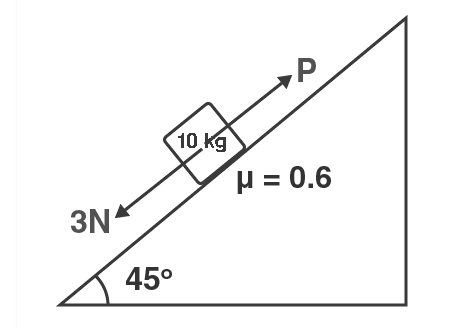1. a) 64 N
2. b) 32 N
3. c) 12 N
4. d) 3 N

Solution:

1. Upward force = Downward force

mg sinθ +3 = p + l

mg sinθ +3=P + µ(N)

substituting,

[(10 x 10)/√2] + 3= P + [(0.6 x 10 x10)/√2]

Simplifying P = 32N

Question 2. For path ABC, Heat given to the system is 60 J and work done by the system is 30 J. For path ADC, work done by the system is 10J. The heat given to the system for path ADC is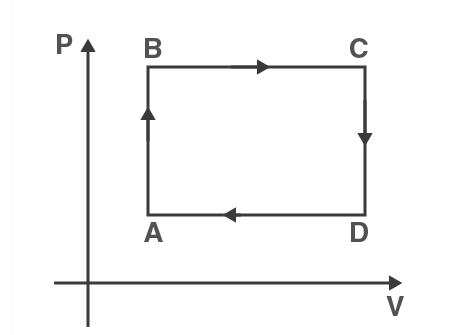1. a) 100 J
2. b) 80 J
3. c) 40 J
4. d) 60 J

Solution:

1. Change in Int energy should be same

ΔU = 60 - 30 = 30

In process ABC

ΔQ = ΔU + Δw

= 30 + 10 = 40 J

Question 3. Initially, an object is kept at a distance of 10 cm from the convex lens and a sharp image is formed at 10 cm ahead of the lens on the screen. Now a glass plate of µ = 1.5 cm and thickness 1.5 cm is placed between object and lens. The distance by which the screen be shifted to get a sharp image on the screen will be: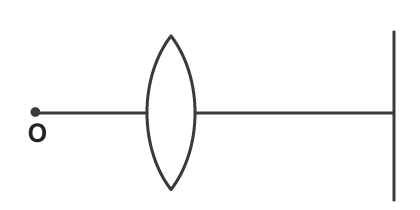1. a) 1.1 cm
2. b) 0.55 cm away from the lens
3. c) 0.55 cm towards the lens
4. d) 5 cm

Solution:

1. 2f ⇒ f= 5cm

S = t (1 – (1/µ)) = 1.5(1 – (1/1.5)) = 0.5

along incident rayS is positive

new object distance = 10 -0.5 = 9.5

(1/v) = (1/u) + (1/f)

V =uf/(u+f) = (5 x 9.5)/(9.5 – 5) = 10.55 cm

The screen should be shifted by a distance 0.55 cm away from the lens

Question 4. A planet of mass m having angular momentum L is revolving around the sun. The aerial velocity of the planet will be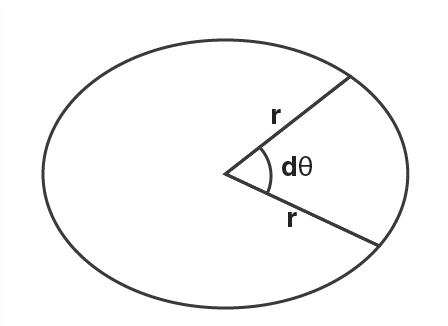1. a) L/m
2. b) L/2m
3. c) 2L/m
4. d) L/4m

Solution:

1. For small dθ,

dA = (1/2) r2

(dA/dt) = = (1/2) r2 dθ = (1/2) ) r2 w = L/2m (since L = m/ r2 w)

Question 5. The velocity of a particle v at any instant is $\vec{v}=y\hat{i}+x\hat{j}$. The equation of trajectory of the particle is:

1. a) x 2 + y2 = constant
2. b) y 2 = x 2 + constant
3. c) xy = constant
4. d) None of these

Solution:

1. $\vec{v}=y\hat{i}+x\hat{j}$

(dx/dt) = y

xdx = ydy

dy/dx

$\frac{x^{2}}{2}+\frac{y^{2}}{2}=C$

x2- y2 = K

Question 6.Initially block of mass M is at rest on a frictionless floor and the spring is in relaxed condition A constant force is applied on the block as shown in the figure. The maximum velocity of block is: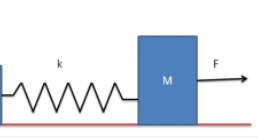1. a) F/√mK
2. b) 2F /√mK
3. c) F/2√ mK
4. d) F √2mK

Solution:

1. Maximum speed is at equilibrium where

F=kx⇒x= F/k

​Now, F⋅x= (1/2)mv2 + (1/2)kx2

F⋅(F/k)= (1/2)mv2 + (1/2)k(F/k)2

Solving we get,

v= F/√mK

Question 7. Magnetic field at point O is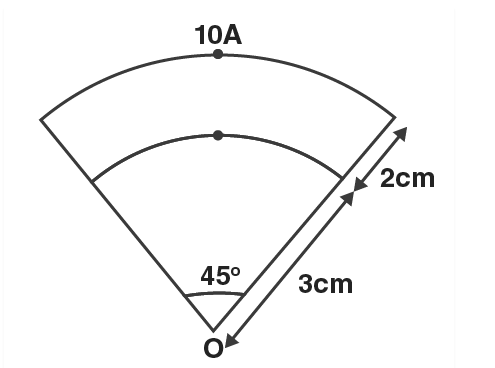1. a) 1.5 x 10- 5
2. b) 10- 5
3. c) 2 x 10- 5
4. d) 10- 4

Solution:

1. Solution:

B0 = BAB + BBC + BCD + BOA

= (µ0Iθ/4πrBC) + 0 + (µ0Iθ/4πrOA)

= Substituting values we get BO = 10-5

Question 8. Charge Q is uniformly distributed over a ring of radius R. The height h, on the axis of the ring at which electric field is maximum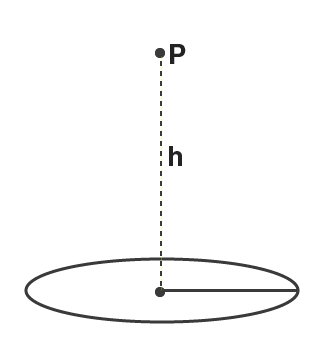1. a) R /√2
2. b) R/2
3. c) R
4. d) None of these

Solution:

1. E (along) axis of circular coil = kQh/(h2+R2)3/2

for max value of E (dE/dh) =0

Differentiating E w.r.t.h and equating it to O

we get h = ±R/√2

Question 9.Two radioactive elements A & B have initially activity 10 curie & 20 curie respectively. If A has twice the no. of moles as that of B. The decay constant λA & λB can be;

1. a) (10, 5)
2. b) (5, 20)
3. c) (20, 10)
4. d) (50, 100)

Solution:

1. Conceptual

λN = A

$\frac{\lambda _{A}}{\lambda _{B}}\frac{N_{A}}{N_{B}}=\frac{A _{A}}{A_{B}}$

$\frac{N_{A}}{N_{B}}=2$

$\frac{\lambda _{A}}{\lambda _{B}}(2)= \frac{10}{20}$

$\frac{\lambda _{A}}{\lambda _{B}}= \frac{5}{20}$

Question 10. A conducting loop of resistance 10Ω and area 3.5 x 10 -3m 2 is placed in uniform and time varying magnetic field B = 0.4 sin (50πt). The Charge passing through the loop in t = 0 to t = 10 ms is:

1. a) 140 µC
2. b) 70 µC
3. c) 280 µC
4. d) 100 µC

Solution:

1. $Q=\frac{\Delta \phi }{R}$

$\varepsilon =\frac{d \phi }{dt}$

$iR =\frac{d \phi }{dt}$

$\frac{dQ}{dt}R=\frac{d \phi }{dt}$

$\int dQ =\int Rd \phi$

$Q=\frac{1}{R}\int dQ$

$Q=\frac{1}{R}3.5 \times 10^{-3}\int_{0}^{10ms}0.4sin(50\pi t)dt$

= 140 µC

Question 11. If current in a current carrying wire is 1.5A, number of free electrons per unit volume is 8 x1028m3 and area of cross section is 5 mm2 . Drift velocity of electrons will be

1. a) 0.02 mm/s
2. b) 2 mm/s
3. c) 0.2 mm/s
4. d) None of these

Solution:

1. i = e n A Vd (relation between i and Vd)

$V_{d}=\frac{1}{enA}= \frac{1.5}{1.6 \times 10^{-19}\times 5 \times10^{-6} \times 8 \times 10^{28}}=0.02$

Question 12. If temperature difference T1 – T2 is 120°C. The temperature difference between points A & B is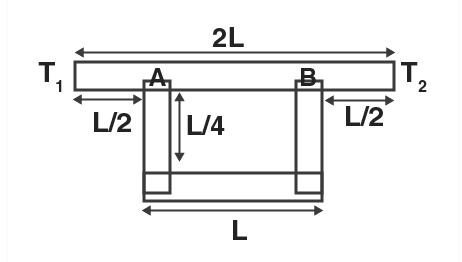1. a) 30
2. b) 45
3. c) 60
4. d) 75

Solution:

1. Effective resistance between A and B is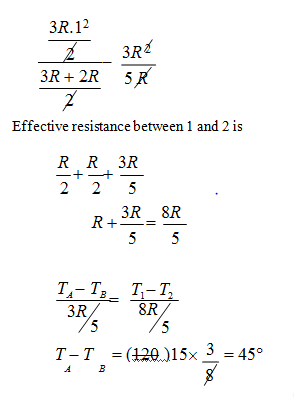Question 13.Three blocks m, m and M are kept on a frictionless floor as shown in figure. The left most block is given velocity v towards right. All the collisions between the blocks are perfectly inelastic. The loss in kinetic energy after all the collisions is 5/6th of initial kinetic energy. The ratio of M/m will be: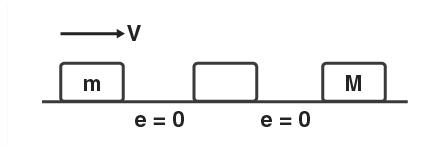1. a) 1/8
2. b) 1/4
3. c) 4
4. d) 2

Solution:

1. $\frac{mu}{2m+M}= V_{f}$

$K_{f} = \frac{1}{6}K_{1}$

$\frac{1}{2} \frac{P^{2}}{2m+M}= \frac{1}{6}\frac{1}{2}\frac{P^{2}}{m}$

6m = 2m+M =4m = M

Question 14. In mixture 2 mole of He and 1 mole of Ar is present. Find $\frac{(V_{RMS})_{He}}{(V_{RMS})_{Ar}}$ at 300 K.

1. a) 6.32
2. b) 1.58
3. c) 3.16
4. d) 10

Solution:

1. $V_{RMS}= \sqrt{\frac{3RT}{M}}$

$\frac{(V_{RMS})_{He}}{(V_{RMS})_{Ar}} = \sqrt{\frac{M_{Ar}}{M_{He}}}=\sqrt{\frac{40}{4}}=\sqrt{10}$

= 3.16

Question 15. Light of wavelength λ1 = 340 nm and λ2 = 540 nm are incident on a metallic surface. If the ratio of the speed of the electrons ejected is 2. The work function of the metal is

1. a) 1 eV
2. b) 1.85 eV
3. c) 1.5 eV
4. d) 2 eV

Solution:

1. $\frac{hc}{\lambda _{1}}=\phi +\frac{1}{2}m(mv)^{2}$----------(1)

$\frac{hc}{\lambda _{2}}=\phi +\frac{1}{2}(mv)^{2}$-------------(2)

Substituting (2) in (1) we get

hc/λ1 = Φ + 4(hc/λ2 - 4)

3 Φ = 4(12375/5400) - 12375/3400

Φ = 1.838

Question 16. Value of i1 (Ampere) when switch is closed is.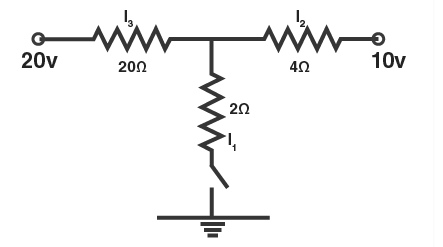1. a) 2
2. b) 5
3. c) 10
4. d) 1

Solution:

1. (V0-20)/2= (V-10)/4 = (V- 0)/2

V=10 , i=10/2 = 5A

Question 17. If net force on charge kept at O is zero. The value of charge q is;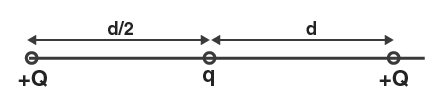1. a) +Q /2
2. b) - Q /2
3. c) + Q/4
4. d) – Q/4

Solution:

1. Net force of q = 0 $\frac{KQq}{(d/2)_{2}}+ \frac{KQq}{(d)^{2}}= 0$

q= - Q/4

Question 18. If the value of electric field E = 6.3 x 1027 volt/m for an electromagnetic wave. The value of magnetic field B will be:

1. a) 5 x 10-19 T
2. b) 2.1 x 1019 T
3. c) 5 x 10-20 T
4. d) 2.1 x 1020 T

Solution:

1. Hint: E/B = C

=> B = E/C

B= 6.3 x 1027 /3 x 108

B= 2.1 x 1019 T

Question 19.Two coherent light sources having intensity I1 and I2. If the ratio of Imax/Imin is 6 : 1. Find I1/I2​​?

1. a) 16/9
2. b) 9/16
3. c) 4/1
4. d) 25/9

Solution:

1. $\frac{I_{max}}{I_{min}}=\frac{(\sqrt{I_{1}}+\sqrt{I_{2}})^{2}}{(\sqrt{I_{1}}-\sqrt{I_{2}})^{2}}$

On Simplifying above expression, we get

(I1/I2) = 25/9

Question 20. If length of resistance wire is increased by 0.5 % keeping the volume constant then change in resistance will be;

1. a) 0 %
2. b) 1 %
3. c) 0.5 %
4. d) 2 %

Solution:

1. $R = \frac{\rho l\times l}{a\times l}=\frac{\rho l^{2}}{V}$

R ∝ l2

$l\omega \times \frac{\Delta R}{I^{2}}=2(\frac{\Delta l}{l}\times 100)$

= 2(0.5)

$100 \times \frac{\Delta R}{I^{2}}=1%$

Question 21. A uniform L shaped rod each of side A is held as shown in the figure. The angle θ such that the rod remains stable will be;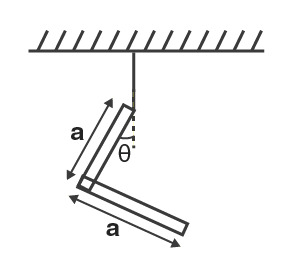1. a) tan-1(1/2)
2. b) tan-1(1/3)
3. c) tan-1(2)
4. d) tan-1(1)

Solution:

1. Clockwise torque = anti clockwise torque

θ = tan-1 (1/3)

Question 22.A rod of acted by two equal forces as shown in the figure. The coefficient of thermal expansion of the rod is α and area of cross section is A. When the temperature the rod increased by Δ t. The length of the rod does not change. The young’s modulus Y will be.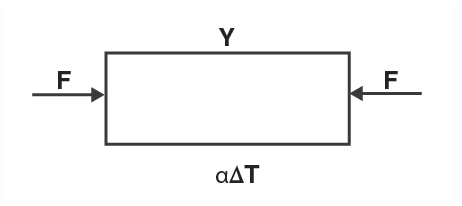1. a) $\frac{F}{2A\alpha \Delta T}$
2. b) $\frac{F}{A\alpha \Delta T}$
3. c) $\frac{2F}{A\alpha \Delta T}$
4. d) $\frac{F}{3A\alpha \Delta T}$

Solution:

1. $\Delta l=l\alpha$ ...(1)

$\Delta l=\frac{Fl}{Ay}$...(2)

From (1) and (2)

y= $\frac{F}{A \alpha T}$

Question 23. A capacitor is formed by two square metal-plates of edge a, separated by a distance d. Dielectric of dielectric constants K is filled in the gap as shown in the figure. The equivalent capacitance is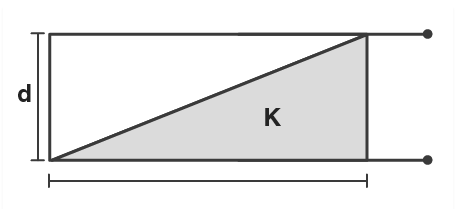1. a) $\frac{k\epsilon _{0}a^{2}lnk}{d(k-1)}$
2. b) $\frac{k\epsilon _{0}a^{2}lnk}{d(k-2)}$
3. c) $\frac{k\epsilon _{0}a^{2}lnk}{2d(k-1)}$
4. d) $\frac{2k\epsilon _{0}a^{2}lnk}{d(k-1)}$

Solution:

1. y/d = x/a …(1)

$dC_{2}=\frac{\epsilon _{0}adx}{d-y}$

$dC_{1}=\frac{K\epsilon _{0}adx}{y}$

$dC_{eq}=\frac{dC_{1}dC_{2}}{dC_{1}+dC_{2}}= \frac{\epsilon _{0}adx}{\frac{C_{1}}{k}+ \frac{d-y}{1}}$

$C_{eq}=\int_{0}^{a}\frac{\epsilon _{0}adx}{\frac{C_{1}}{k}+ \frac{d-y}{1}}$

After integrating, we get option 1 as an answer.

Question 24. In a semiconductor mobility of electron, i.e. drift velocity per unit applied electric field is 1.6 (S.I unit). Density of electrons is 1019/m3. (Neglect holes concentration). Resistivity of semiconductor is:

1. a) 0.4 Ωm
2. b) 2 Ωm
3. c) 4 Ωm
4. d) 0.2 Ωm

Solution:

1. $\sigma =ne\mu \Rightarrow \rho =\frac{1}{ne\mu }=\frac{1}{10^{19}\times 1.6\times 10^{-19}\times 16}=0.4\Omega m$

Question 25.A block of mass M is hanging by a string of negligible mass in a car. The speed of the wave in the string 60 m/s. Now the car is accelerated horizontally by an acceleration at the speed of wave in the string is 60.5 m/s. What is a in terms of g?

1. a) g/5
2. b) g/10
3. c) g/20
4. d) g/30

Solution:

1. Speed of wave on string

$v=\sqrt{\frac{T}{\mu }}$

- wherein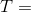Tension in the string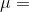linear mass density

$v=\sqrt{\frac{T}{m }}$

$60=\sqrt{\frac{Mg}{m }}$ given m<< M

When the car accelerates with a,

Resultant acceleration = $\sqrt{a^{2}+g^{2}}$

$60=\sqrt{\frac{M\sqrt{a^{2}+g^{2}}}{m }}$

$(\frac{60.5}{60})^{2}=\frac{\sqrt{a^{2}+g^{2}}}{g}$

$(\frac{60.5}{60})^{4}=\frac{{a^{2}+g^{2}}}{g}$

$a^{2}=g^{2}\times (\frac{60.5}{60})^{4}-g^{2}$

a = 0.1837 g

= g/5.44

a=g/5 (approx)

### JEE Main 2019 January Physics Paper with Solutions